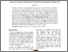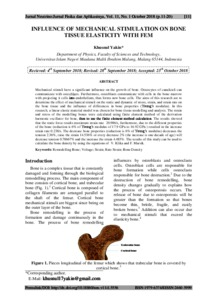# Influence of mechanical stimulation on bone tissue elasticity with fem

Yakin, Khusnul (2018) Influence of mechanical stimulation on bone tissue elasticity with fem. Jurnal Neutrino, 11 (1). pp. 11-20. ISSN 2460-5999Preview
Text
7426.pdf - Published Version

Full text available at: http://ejournal.uin-malang.ac.id/index.php/NEUTRIN...

## Abstract

Mechanical stimuli have a significant influence on the growth of bone. Osteocytes of canaliculi can communicate with osteoblasts. Furthermore, osteoblasts communicate with cells in the bone marrow with projecting it cells into endothelium, thus forms new bone cells. The aims of this research are to determine the effect of mechanical stimuli on the static and dynamic of stress, strain, and strain rate on the bone tissue and the influence of differences in bone properties (Young’s modulus). In this research, a linear elastic material model was chosen for bone tissue modelling and analysis. The strain and stress of the modelling bones were calculated using finite element method of the derivation harmonic oscillator for bone, then to use the finite element method calculation. The results showed that the static force results maximum strain rate 20.986/s, furthermore, due to the different properties of the bone (reduction is 6% of Young’s modulus of 17.9 GPa to 16.92 GPa ) resulted in the increase strain rate 0.238/s. The decrease bone properties (reduction is 6% of Young’s modulus) decreases the tension 2.36%, raise the strain 14.536% or every decrease 2% (the increase is one decade of age) will decrease tension 0.78667% and the increase the strain 4.485%. The results of this study can be used to calculate the bone density by using the equations of V. Klika and F. Marsik.

Item Type: Journal Article remodelling bone; voltage; strain; rate strain; bone density 01 MATHEMATICAL SCIENCES > 0103 Numerical and Computational mathematics > 010302 Numerical Solution of Differential and Integral Equations02 PHYSICAL SCIENCES > 0202 Classical Physics > 020399 Classical Physics not elsewhere classified Faculty of Mathematics and Sciences > Department of Physics Khusnul Yakin 27 Nov 2020 21:14View Item